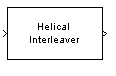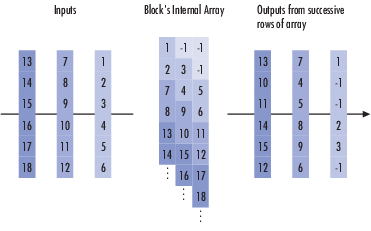Documentation

### This is machine translation

Mouseover text to see original. Click the button below to return to the English version of the page.

# Helical Interleaver

Permute input symbols using helical array

## Library

Convolutional sublibrary of Interleaving## Description

The Helical Interleaver block permutes the symbols in the input signal by placing them in an array in a helical fashion and then sending rows of the array to the output port.

The block uses the array internally for its computations. If C is the Number of columns in helical array parameter, then the array has C columns and unlimited rows. If N is the Group size parameter, then the block accepts an input of length C·N at each time step and partitions the input into consecutive groups of N symbols. Counting from the beginning of the simulation, the block places the kth group in the array along column k mod C. The placement is helical because of the reduction modulo C and because the first symbol in the kth group is in row 1+(k-1)· s, where s is the Helical array step size parameter. Positions in the array that do not contain input symbols have default contents specified by the Initial condition parameter.

The block sends C·N symbols from the array to the output port by reading the next N rows sequentially. At a given time step, the output symbols might be the Initial condition parameter value, symbols from that time step's input vector, or symbols left in the array from a previous time step.

This block accepts a column vector input signal containing C·N elements.

The block can accept the data types `int8`, `uint8`, `int16`, `uint16`, `int32`, `uint32`, `boolean`, `single`, `double`, and fixed-point. The data type of this output will be the same as that of the input signal.

## Parameters

Number of columns in helical array

The number of columns, C, in the helical array.

Group size

The size, N, of each group of input symbols. The input width is C times N.

Helical array step size

The number of rows of separation between consecutive input groups in their respective columns of the helical array.

Initial conditions

A scalar that fills the array before the first input is placed.

## Examples

Suppose that C = 3, N = 2, the Helical array step size parameter is 1, and the Initial condition parameter is -1. After receiving inputs of `[1:6]'`, `[7:12]'`, and `[13:18]'`, the block's internal array looks like the schematic below. The coloring of the inputs and the array indicate how the input symbols are placed within the array. The outputs at the first three time steps are `[1; -1; -1; 2; 3; -1]`, `[7; 4; 5; 8; 9; 6]`, and `[13; 10; 11; 14; 15; 12]`. (The outputs are not color-coded in the schematic.)## Pair Block

Helical Deinterleaver

## References

 Berlekamp, E. R. and P. Tong. "Improved Interleavers for Algebraic Block Codes." U. S. Patent 4559625, Dec. 17, 1985.